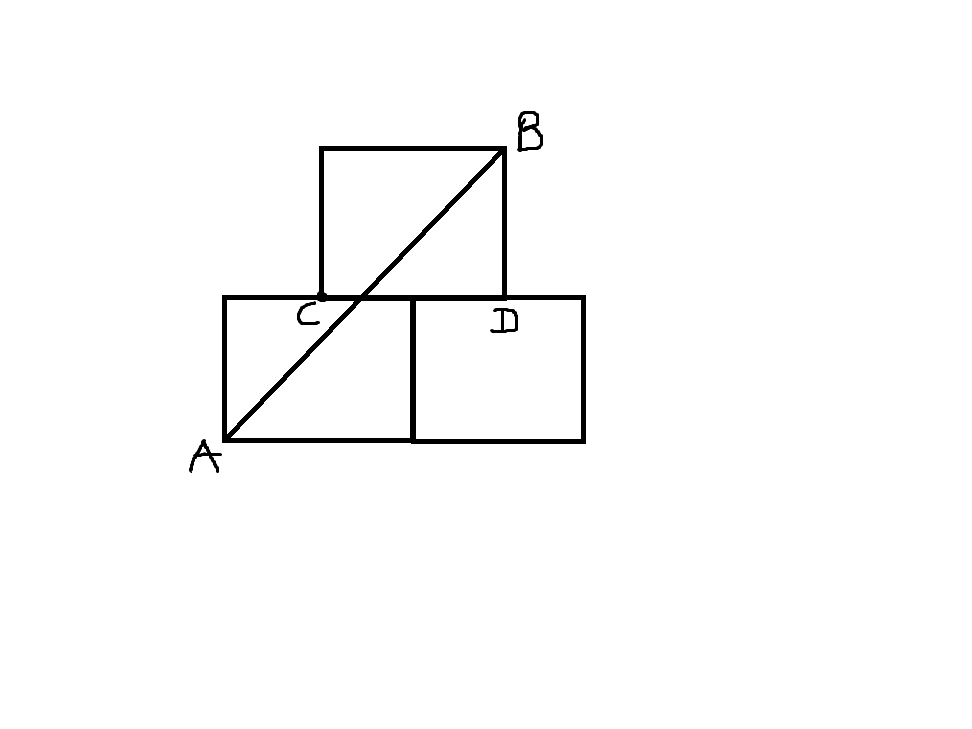# Q 4

Geometry Level 2Three equal squares are kept as in the figure. C, D being the mid points of the respective sides of the lower squares. If AB = 100 cm, Area of each square in $cm^{2}$ is ?

×